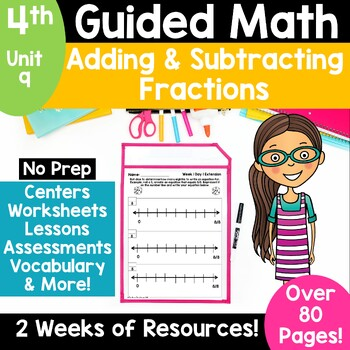Subject
Resource Type
Format
Zip (9 MB|61 pages)
Standards
\$8.50
\$8.50

#### Also included in

1. This is a BUNDLE of my 4th Grade Guided Math Units. There are a total of 15 units.Lesson plans are standards based and have a specific, achievable objective for each day. Units include pre and post assessments, daily practice pages, daily extension activities, vocabulary cards, questioning cards, po
\$108.00
\$136.00
Save \$28.00

### Description

This 4th grade math unit covers adding and subtracting fractions. Standards 4.NF.3 and 4.NF.5 are covered. Your students will learn about adding fractions using picture models and number lines, decomposing fractions into an addition equation, writing improper fractions and mixed numbers, as well as adding improper fractions and mixed numbers. They'll also learn to subtract fractions and solve word problems.

4th Grade Guided Math units are recommended for small group, targeted instruction, but also can be used in a whole group format.

There are two separate PDF's included. One with CC Standards and one with TEKS standards.

What's included:

Vocabulary and Definition Cards

•Questioning Cards

•Printables to support instruction

•2 weeks of adding and subtracting lesson plans (Please note--the links on the lesson plan are to additional products in my store that cover a certain standard. They are NOT necessary to teach the lessons included in Guided Math and are not included with this product.) ⚠️An editable (PowerPoint) version of the lesson plans is now included.

Practice pages (One for each day. Day 5 practice pages are a review of the objectives covered during the week and can be used as assessment.)

Extension activities (One for each day of the week! Use these in one of your centers or another small group rotation!)

Pre and Post Assessments for the unit

Answer keys now included for the assessments and practice pages

~~~~~~~~~~~~~~~~~~~~~~~~~~~~~~~~~~~~~~~~~~~~~

~~~~~~~~~~~~~~~~~~~~~~~~~~~~~~~~~~~~~~~~~~~~~

Total Pages
61 pages
Included
Teaching Duration
N/A
Report this Resource to TpT
Reported resources will be reviewed by our team. Report this resource to let us know if this resource violates TpT’s content guidelines.

### Standards

to see state-specific standards (only available in the US).
Express a fraction with denominator 10 as an equivalent fraction with denominator 100, and use this technique to add two fractions with respective denominators 10 and 100. For example, express 3/10 as 30/100, and add 3/10 + 4/100 = 34/100.
Solve word problems involving addition and subtraction of fractions referring to the same whole and having like denominators, e.g., by using visual fraction models and equations to represent the problem.
Add and subtract mixed numbers with like denominators, e.g., by replacing each mixed number with an equivalent fraction, and/or by using properties of operations and the relationship between addition and subtraction.
Decompose a fraction into a sum of fractions with the same denominator in more than one way, recording each decomposition by an equation. Justify decompositions, e.g., by using a visual fraction model. Examples: 3/8 = 1/8 + 1/8 + 1/8; 3/8 = 1/8 + 2/8; 2 1/8 = 1 + 1 + 1/8 = 8/8 + 8/8 + 1/8.
Understand addition and subtraction of fractions as joining and separating parts referring to the same whole.